## Blog

### Mesh Convergence: The modern way

Many engineers in the beginning of their career do not care about what mesh size they are using. Sometimes a look-alike component is used as a guide for generating mesh without any reference to the loading conditions and effect on accuracy of results. Usually if a finite element analysis completes successfully and gives an interesting result, it is considered sufficient. But the element sizes certainly have an influence on the accuracy of FEA results. A mesh convergence study is performed to make sure that element sizes are sufficient such that the solution obtained using finite element analysis is accurate to a desired level. What strategy should be used to refine mesh is always an important question. In this blog we are going to discuss the traditional and modern way of refining mesh with aim to reduce the error in solution. We will look at two cases and see how the traditional and modern way work for these cases. The ‘modern’ way is really not modern in the true sense. I call it modern because it is not frequently used although many FEA software offer such capabilities.

### Case 1

Let us consider a cantilever beam shown in Figure 1. The beam is fixed on the left side and a force is applied on right end. The applied force is distributed along the length of the edge which has a magnitude of 10 kN/m. The beam has a length of 1m and cross section of 300mm x 20mm. The load is applied slowly such that inertia effects can be neglected. So analysis can be performed using the static procedure.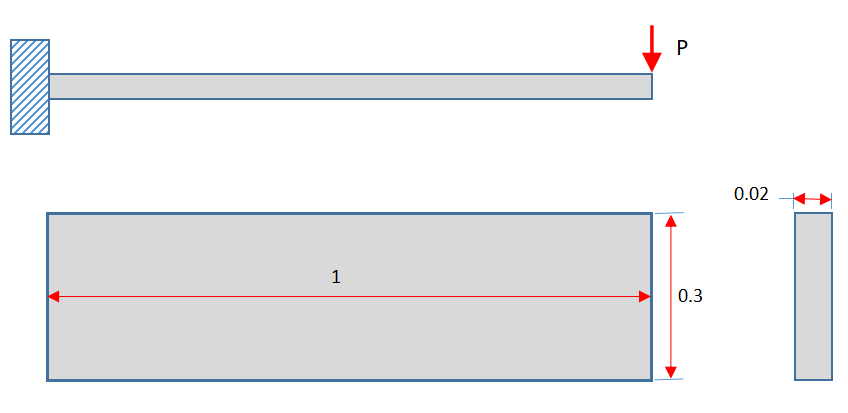Analytical solution of the problem is shown below. A maximum value of bending stress (the stress normal to cross section) of 150 MPa is computed using the analytical relation.The purpose of choosing a simple problem for the study is to show how the mesh density affects the numerical solution which can be compared to analytical results. For numerical simulation some assumptions have been made to obtain smooth stress plot. We will not discuss them here instead focus on the subject of mesh convergence. To make the discussion more focused only four-node shell elements (S4 in Abaqus) will be considered.

The results for the analysis of the cantilever beam are shown in the Figure 2. In this analysis six elements are used along the length of the beam. A maximum stress of 125.5 MPa occurs. This value is significantly lower than the analytical solution.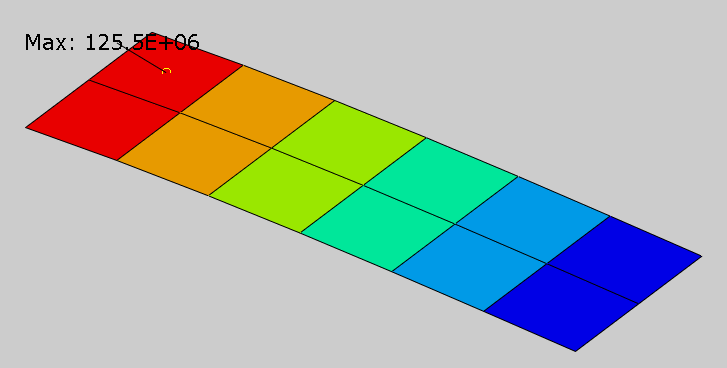Figure 2: Contour plot of bending stress for coarse mesh

Now we will execute more analysis jobs using finer mesh densities and see how it affects the solution. The Figure 3 shows the contour plot of bending stress for finer mesh densities. It can seen that as the number of elements increase, maximum bending stress approaches the value obtained using analytical relation.Figure 3: Mesh refinement using global mesh size. Bending stress is plotted in all cases.

It is customary to plot the quantity of interest against the number of elements during a convergence study. In the Figure 4 maximum bending stress is plotted against the number of elements in the beam. It can be seen that as more elements are used to mesh the beam, the predicted value of maximum stress approaches the analytical value. Notice that after point A, mesh refinement produces negligible change in the maximum stress value. At point A, the beam has 30 elements and gives a value of 147.5 MPa of maximum bending stress. At point E, the beam has 3000 elements and gives a value of 149.3 MPa of maximum bending stress. Although this value is very close to the value obtained from analytical solution, the computational cost is too high to justify such a refined mesh. The mesh is said to be converged when further mesh refinement produces no/negligible change in the solution. So we can say that mesh converges at point A. As we keep on increasing the elements, the problem requires more and more computational resources. So we need to consider both the computational cost and desired accuracy while generating a mesh. Before performing a detailed analysis, a mesh convergence study is recommended to be performed to determine how small the elements need to be to ensure that the finite element solution is not affected by changing the size of the mesh.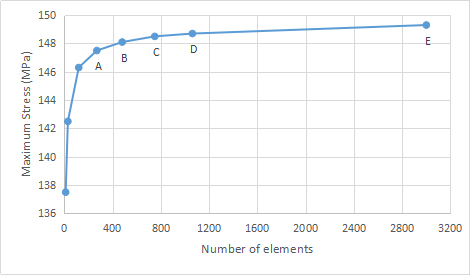Figure 4: Convergence of stresses using global mesh refinement

### Case 2

Now consider a thick cylinder shown in the Figure 5. A thick cylinder is cylinder whose wall thickness is greater than 1/20 times of its internal diameter. We assume Ri = 0.1 m, Ro = 0.4 m and an internal pressure of 100 MPa. The cylinder is assumed to be made from steel with a Young’s modulus of 200 GPa and a Poisson’s ratio of 0.3. It will be meshed with linear plane strain elements.Figure 5: Schematic illustration of thick cylinder

By taking advantage of the symmetry, only 45-degree sector of the cylinder will be analyzed as shown in the Figure 6. Symmetry boundary conditions will be applied on the symmetric edges of the sector. A coarse mesh as shown in the figure will be used for the initial analysis.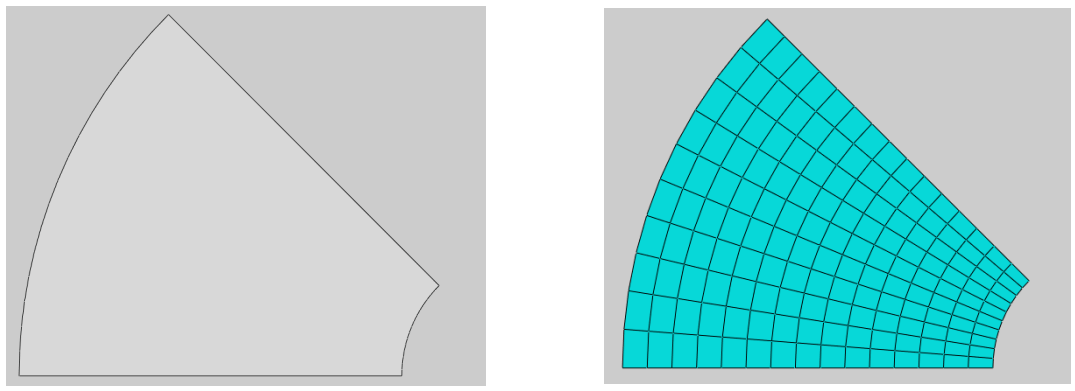Figure 6: Sector of the thick cylinder considered for analysis

Radial and circumferential stresses are the variables of utmost interest in the analysis. Here we will only discuss the radial stresses in the cylinder. The contour plot of radial stresses is shown in the Figure 7 when an analysis is performed using the coarse mesh.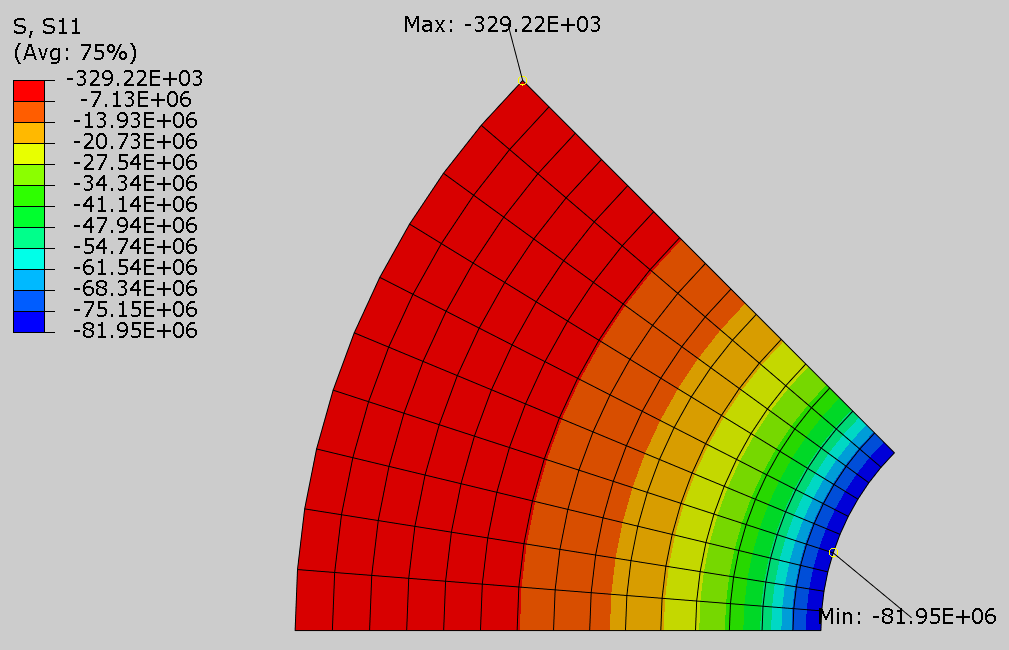Figure 7: Contour plot of radial stresses

The contour plot shows the maximum radial stress magnitude of 81.95 MPa at the inner radius of cylinder. The analysis results seem reasonable but we are not sure how accurate is this solution. In the previous case, a convergence study was performed by refining the mesh throughout the modeling domain and a curve was plotted highlighting the relation between maximum stress and number of elements. In the current case we are going to plot contour of an error indicator to have an idea of the accuracy of the solution. An error indicator output variable provides an insight of the extent and spatial distribution of the discretization error in the solution. When we know the distribution of discretization error, we can refine the mesh accordingly.

The contour plot of the Mises stress error indicator (MISESERI in Abaqus) at the end of analysis is shown in the Figure 8. In simple words this indicator gives an estimation of error in the Mises stress. It can be seen that the maximum error of 13.45 MPa is predicted to occur near the internal radius of the cylinder. The error in the solution decreases as the distance from center of the cylinder increases. The lowest value of 0.367 MPa of error indicator occurs near outer radius. With this information in hand it can be concluded that we need to refine mesh in the region close to internal radius to accurately predict the maximum stresses.Figure 8: Contour plot of error indicator

The discretization error estimation provides guidance for mesh redesign. The extent and spatial distribution of the discretization error in a finite element solution helps to determine where to refine or coarsen a mesh. A FEA software can compute the discretization error automatically without any additional input from the analyst. The error is estimated based on the assumption that the solution should be continuous over the elements and that jumps in the stresses are an indication of discretization error. A smoothed stress value by averaging the stresses between the elements at the nodes is computed. It is assumed that the smoothed solution more closely approximates the exact solution than does the finite element solution. The discretization error is estimated as a difference between the finite element solution and the smoothed approximation.

As the error is highest near the internal radius and decreases with increasing radius, a new mesh is generated such that element density is highest close to internal radius and decreases progressively outwards. The new mesh is shown in the Figure 9.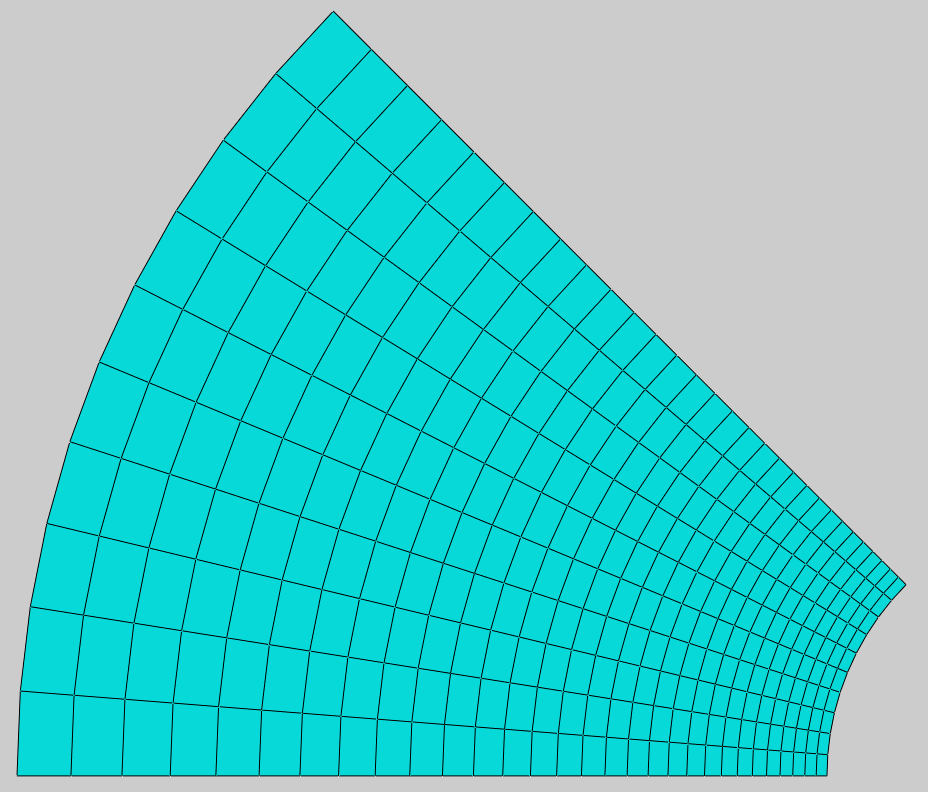Figure 9: New mesh design of cylinder

The contour plot of radial stress when the new mesh is used for analysis is shown in the Figure 10. It shows the maximum radial stress magnitude of 95.59 MPa at the inner radius of cylinder. This is a considerable increase as compared to the coarse mesh.Figure 10: Contour plot of radial stresses for refined mesh

The contour plot of the Mises stress error indicator at the end of analysis is shown in the Figure 11. It can be seen that the maximum error of 3.99 MPa is predicted to occur at the internal radius of the cylinder. This is a considerable decrease as compared to the error estimate of 13.45 MPa for coarse mesh. The error in the solution decreases as the distance from center of the cylinder increases. The lowest value of 0.365 MPa of error indicator occurs at outer radius. If we need to reduce the error further, we can refine the mesh in the region close to internal radius.Figure 11: Contour plot of error indicator after refining mesh

### Comparing to Analytical Results

For the case of internal pressure applied to a thick-walled cylinder, the radial stresses can be computed using the following relation: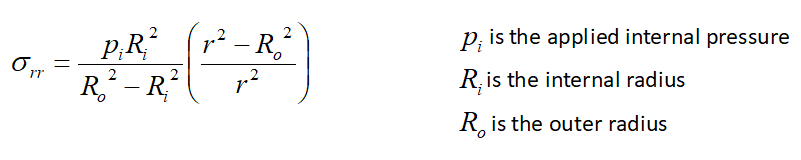This relation gives a magnitude of 100 MPa at inner radius. So we know that the value of radial stress of 95.59 MPa obtained using refined mesh is close to the exact solution and if the mesh is further refined, finite element analysis results come even closer to analytical results. We do not always have luxury of comparing FE results to analytical solution, in such cases contour plot of error indicator proves really helpful in deciding where mesh refinement is needed.

### Concluding remarks

Mesh density affects the accuracy of predictions made by a finite element analysis significantly. To find the optimal size of mesh elements, a mesh convergence study should be performed. Traditionally such studies are performed by refining mesh size globally. Although it is very handy to implement, this strategy results in models with large number of elements. As an alternative,  distribution of discretization error can be used as a guide to refine mesh in the regions where error is highest. This results in models with fewer elements as compared to global mesh refinement strategy. This is also the basis of adaptive meshing which will be covered in next blog.

If you want to learn about mesh convergence and related topics in depth, you could have a look at the online course “Solving Non-linear Problems with Abaqus